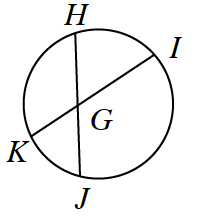### Home > INT2 > Chapter 11 > Lesson 11.1.3 > Problem11-33

11-33.$\overline{HJ}$ and $\overline{IK}$ intersect at $G$. If $HG = 9$, $GJ = 8$, and $GK = 6$, what is $IG$?

$\triangle KGJ \sim \triangle HGI$

$IG = 12$